# 0708-1300/Class notes for Thursday, January 24

(diff) ← Older revision | Latest revision (diff) | Newer revision → (diff)
Announcements go here

## Typed Notes

The notes below are by the students and for the students. Hopefully they are useful, but they come with no guarantee of any kind.

Proof of Van Kampen

Let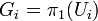$G_i = \pi_1(U_i)$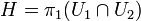$H = \pi_1(U_1\cap U_2)$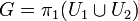$G=\pi_1(U_1\cup U_2)$

We aim to show that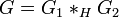$G=G_1*_H G_2$

Hence, we want to define two maps: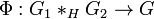$\Phi:G_1*_H G_2\rightarrow G$ and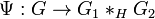$\Psi:G\rightarrow G_1*_H G_2$

such that they are inverses of each other.

Now, recall the commuting diagram: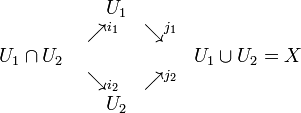$\begin{matrix} &\ \ \ \ U_1&&\\ &\nearrow^{i_1}&\searrow^{j_1}&\\ U_1\cap U_2&&&U_1\cup U_2 = X\\ &\searrow_{i_2}&\nearrow^{j_2}&\\ &\ \ \ \ U_2&&\\ \end{matrix}$

Further, let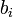$b_i$ alternate between 1 and 2 for successive i's.

Hence we define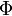$\Phi$ via for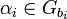$\alpha_i\in G_{b_i}$,

[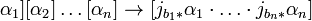$\alpha_1][\alpha_2]\ldots[\alpha_n]\rightarrow [j_{b_1 *}\alpha_1\cdot\ldots\cdot j_{b_n *}\alpha_n]$

Clearly this is well defined. We need to check the relations in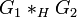$G_1*_H G_2$ indeed hold. Well, the identity element corresponds to the identity path so the relation that removes identities holds. Furthermore, the concatenation of paths is a sum after$\Phi$ and the definition necessitates the third relation holds.

Now for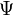$\Psi$:

Elements in G correspond with paths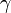$\gamma$ in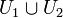$U_1\cup U_2$

On consider such a$\gamma$. The Lesbegue Lemma let us break$\gamma$ up so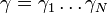$\gamma = \gamma_1\ldots\gamma_N$ such that each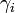$\gamma_i$ is in just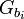$G_{b_i}$

Now,$\gamma_i$ does not go from base point to base point, so we can't consider it as a loop itself. Let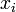$x_i$ denote the endpoint of$\gamma_i$ (and hence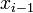$x_{i-1}$ is the beginning point) and further,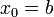$x_0 = b$ the base point.

Choose paths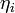$\eta_i$ connecting$x_i$ to b such that if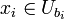$x_i\in U_{b_i}$ then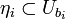$\eta_i\sub U_{b_i}$

Hence,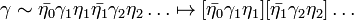$\gamma\sim\bar{\eta_0}\gamma_1\eta_1\bar{\eta_1}\gamma_2\eta_2\ldots \mapsto [\bar{\eta_0}\gamma_1\eta_1][\bar{\eta_1}\gamma_2\eta_2]\ldots$

where each section is entirely in$G_{b_i}$. This above mapping is$\Psi$

We need to show that$\Psi$ is well defined. I.e.,

1)$\Psi$ is independent of the subdivision

2)$\Psi$ is independent of choice of the$\eta_i$'s

3) If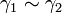$\gamma_1\sim\gamma_2$ then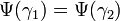$\Psi(\gamma_1) = \Psi(\gamma_2)$

For 1) it is enough to show that one can add or remove a single subdivision point. Suppose you add a new subdivision point c in between two others a and b. Traveling between a and b is the same as going from a to c then to the basepoint and back and then continuing on to b. But this is precisely what happens when you add a subdivision point. Likewise for removing points.

For 2), Suppose$\eta_i$ goes from the basepoint to$x_i$. Add a subdivision point y right beside$x_i$ and remove the one at$x_i$. Then add again the original point$x_i$. The trick is that the new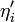$\eta_i'$ is the one used when adding y. The map from the new$x_i$ to the basepoint is this$\eta_i'$ with the infinitesimal connection between y and$x_i$ added. The subdivision point y is then removed leaving our original configuration of basepoints, only with the path from$x_i$ to b now being$\eta_i'$ instead of the original$\eta_i$

For 3) we consider the homotopy between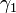$\gamma_1$ and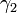$\gamma_2$ thought of as a square with$\gamma_1$ on the bottom and$\gamma_2$ on the top. We use the Lesbegue Lemma to subdivide the square into many subsquares such that each one is entirely in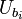$U_{b_i}$

We further modify the homotopy H to a new homotopy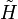$\tilde{H}$ such that each grid point gets mapped to b.

This can be thought of as "pinching" each gird point and pulling it to b or, alternatively, as tossing a "handkerchief on a bed of nails"

If we let$\gamma_1$ be broken in to section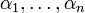$\alpha_1,\ldots, \alpha_n$ along the bottom then$\tilde{H}$ first lifts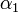$\alpha_1$ to the top and right sides of the subsquare such that the gridpoint goes to b.$\tilde{H}$ then moves the next square up in an analogous manner until we are at the top with$\gamma_2$.